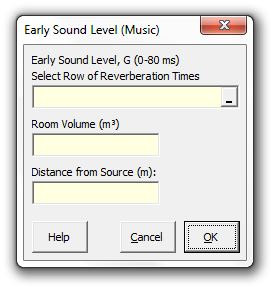### Strutt Help

Early Sound Level (Music)    1/1, 1/3

Strutt|Auditorium Acoustics|Early Sound Level (Music) calculates the early G_(0-80ms) component of the sound field in an auditorium using Barron's revised theory.Revised theory accounts for the decrease in the strength of the reflected sound with distance which occurs with distance in large(r) auditoria - i.e. the reverberant sound component is not constant when using revised theory, but decays exponentially with distance from the source.

The calculation assumes an omnidirectional point source and diffuse conditions in the auditorium.

Note that the resulting level is expressed as strength (G) and can be converted to 'relative loudness' (i.e. relative to the source L_W) by subtracting 31 dB.

The direct sound is calculated using the following relationship:

d = 100/r^2, where

r is the distance from the source.

The early reflections (up to 80 ms) are calculated using:

e=31200/V e^(-(0.04 r)/T) (1-e^(-1.11/T))
, where

V is the volume of the auditorium.
T is the reverberation time.

Strutt adds together the direct and early reflection components to get the total early sound level:

G_(0-80ms)=10log(d+e)

Reference: Barron Auditorium Acoustics and Architectural Design, 2nd edition, Appendix B.3